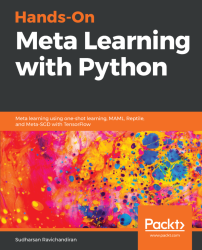•#### Hands-On Meta Learning with Python#### Overview of this book

Meta learning is an exciting research trend in machine learning, which enables a model to understand the learning process. Unlike other ML paradigms, with meta learning you can learn from small datasets faster. Hands-On Meta Learning with Python starts by explaining the fundamentals of meta learning and helps you understand the concept of learning to learn. You will delve into various one-shot learning algorithms, like siamese, prototypical, relation and memory-augmented networks by implementing them in TensorFlow and Keras. As you make your way through the book, you will dive into state-of-the-art meta learning algorithms such as MAML, Reptile, and CAML. You will then explore how to learn quickly with Meta-SGD and discover how you can perform unsupervised learning using meta learning with CACTUs. In the concluding chapters, you will work through recent trends in meta learning such as adversarial meta learning, task agnostic meta learning, and meta imitation learning. By the end of this book, you will be familiar with state-of-the-art meta learning algorithms and able to enable human-like cognition for your machine learning models.
Title PageDedicationContributorsPrefaceFree Chapter
Introduction to Meta LearningFace and Audio Recognition Using Siamese NetworksPrototypical Networks and Their VariantsRelation and Matching Networks Using TensorFlowMemory-Augmented Neural NetworksMAML and Its VariantsMeta-SGD and ReptileGradient Agreement as an Optimization ObjectiveAssessmentsOther Books You May EnjoyIndex## Matching networks

Matching networks are yet another simple and efficient one-shot learning algorithm published by Google's DeepMind team. It can even produce labels for the unobserved class in the dataset.

Let's say we have a support set, S, containing K examples as. When given a query point (a new unseen example),, the matching network predicts the class ofby comparing it with the support set.

We can define this as, whereis the parameterized neural network,is the predicted class for the query point,, andis the support set.will return the probability ofbelonging to each of the classes in the dataset. Then, we select the class ofas the one that has the highest probability. But how does this work exactly? How is this probability computed? Let's us see that now.

The output,, for the query point,, can be predicted as follows:Let's decipher this equation.andare the input and labels of the support set.is the query input— the input to which we want to predict the label....